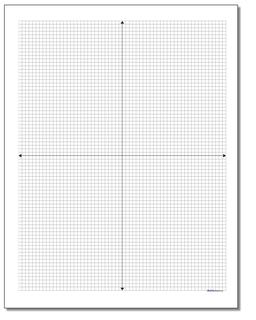# Math Worksheets: Coordinate Plane: Coordinate Plane: Cartesian Standard Graph Paper (Third Worksheet)## Cartesian Standard Graph Paper (Third Template)

PropertyValue
DescriptionCartesian Standard Graph Paper: Printable coordinate planes in inch and metric dimensions in multiple sizes, great for scatterplots, plotting equations, geometry problems or other similar math problems. These are full four-quadrant coordinate planes, blank without axis numbering. Flashing back to 7th grade geometry class? This is the graph paper page for you. Standard sizes 1/4 inch, 1/5 inch, 1/8 inch and 1/10 inch grids . Metric sizes 1 centimeter, 5 millimeter, 2.5 millimeter and 2 millimeter grids. (Third Template)
Resource TypeTemplate